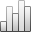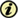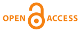More Statisticssince 05 February 2011 :
View(s): 773 (3 ULiège)

### Prediction of Diabetes by Using Artificial Neural Network, Logistic Regression Statistical Model and Combination of Them

Article#### Attached document(s)

Version PDF originale

#### Abstract

Diabetes is a common disease in the world that has not found a cure for it. Annually in our country cost a lot to care for disabilities caused by diabetes, as predicted to treatment, thus more accurately predict the condition of patients is of utmost importance and to forecasts of high precision and reliability must be accurate and to be used the reliable methods. One of these methods using artificial intelligence systems and in particular, is the use of neural networks. Given that the statistical models like logistic regression model are accurate, so in this paper, tried by combining these statistical models and neural networks, create a new compound that has at least error and maximum reliability and is analyzed. With the above suggestions model and different experiences and comparing, numerical results obtained, the accuracy and efficiency of the method has been investigated and acceptable results compared to the neural network and logistic regression methods were obtained. In this research, the criteria are the performance to minimize the error function in neural network training using a neural network in a hybrid model which eventually came to the conclusion that the error function of the neural network is equal to 0.1 and combined neural network model is equal to 0.0002.

Keywords : combined neural network, diabetes, logistic regression, neural network, prediabetes